# Network Analysis June 2010

Note: Answer any FIVE full questions, selecting at least TWO questions from each part.

PART-A

1 a. Using Y-A transformation, find an equivalent resistance between A and B for the network shown in Fig.Q 1(a).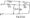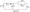b.  For the network shown in Fig.Q 1(b), find the current through 4Q and 60 resistors. (Use mesh analysis).

c.   By using the nodal analysis, find the voltage Vab for the network shown in Fig,Ql(c).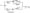2 a. For the network shown in Fig.Q2(a), write the Tie set matrix and obtain the network equilibrium equations in matrix form, using KVL, Calculate the loop currents and branch voltages. Choose AD, BD and CD as tree branches (4, 5, 6 branches).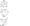Fig. Q 2(a)

b.  For the network shown in Fig.Q2(b), write the f-cutset matrix and hence obtain the equilibrium equations on node basis. Choose AC and BC as twigs.

c. For the network shown in Fig.Q2(c), draw the dual network.

3 a.  State and explain (i) Reciprocity theorem (ii) Millman’s theorem as applied to electrical circuits.

b.  By using superposition principle, find the current through the (4+j3) impedance as shown in Fig.Q3(b).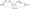4 a. Find the current in the 10£1 resistor in the network shown in Fig.Q4(a), by using Thevenin’s theorem.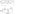b.  For the network shown in Fig.Q4(b), find the Thevenin’s voltage, short circuit current and determine the actual current flowing through the 6Q resistor.

c.   Find the maximum power transferred to the load Zl of the network shown in Fig,Q4(c).

PART-B

5 a. Determine i) the line current, ii) the power factor and iii) the voltage across the coil, when a coil of resistance 40Q and inductance of 0.75H forms a part of a series circuit, for which the resonant frequency is 55Hz, if the supply is 250V, 50Hz.

b.  Give the comparison between the series resonance and parallel resonance.

c.   Derive an expression for the resonance frequency of a resonant circuit consisting of RlL in parallel with RcC. Draw the frequency response curve of the above circuit, indicating the half power frequencies.

6  a. In the network shown in Fig.Q6(a), K is changed from position a to b, at t = 0. Solve . di t d2i ^ for i, — and —- at t = 0+, if R = dt dtthe capacitor is initially uncharged. for i, — and at t = 0+, if R = 1000ft, L = 1 H, C = 0.1 uF and V = 100V. Assume that dt dt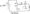b. The network shown in Fig.Q6(b), has two independent node pairs; of the switch K is opened at t = 0, find the following quantities at t – 0+.

i) V   ii) V2      iii) dvj/dt          iv) dv2/dt          v) dii/dt

7 a. In the network shown in Fig.Q7(a), the switch 4K’ is closed and the steady state is reached. At t = 0, the switch is opened. Find the expression for the current in the inductor using Laplace transform.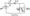b. For the series RLC circuit shown in Fig.Q7(b), the initial conditions are iL0 – 2 A and Vco~2V. It is connected to a DC voltage of 5V at t = 0. Find the current i(t) after the switching action, using Laplace transform.

8 a. The bridged T-RC netv/ork is shown in Fig.Q8(a). For the values given, find the Y and Z parameters.

b. For the network shown in Fig.Q8(b), determine the ABCD parameters.